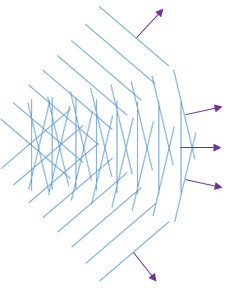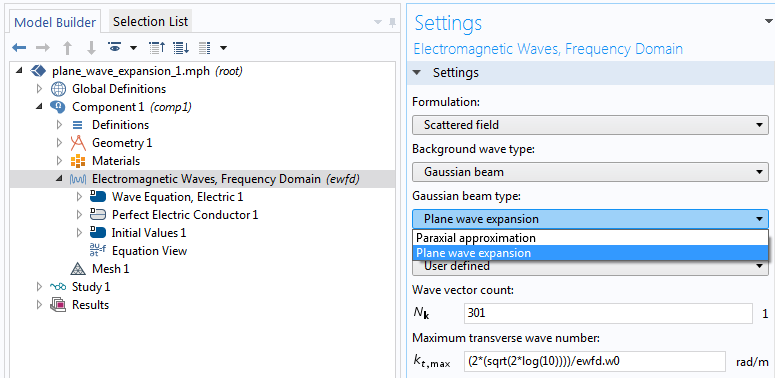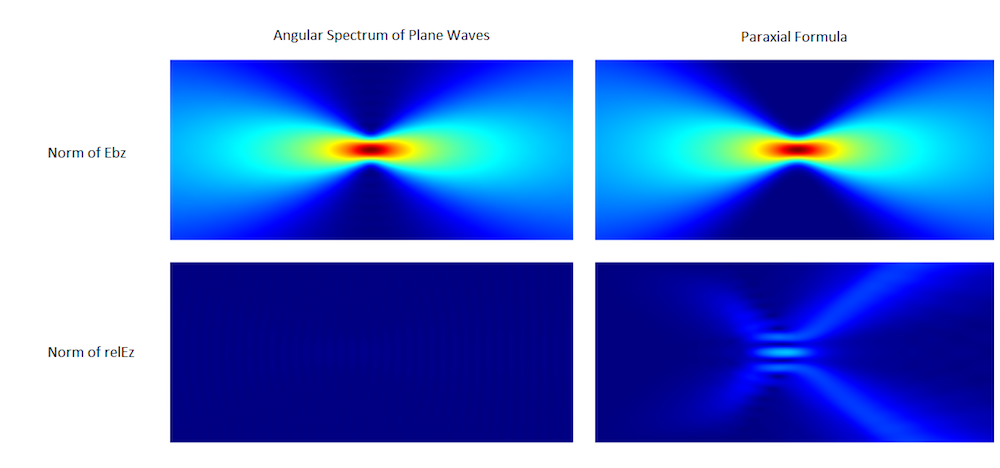# 模拟波动光学的非近轴高斯光束公式

2018年 6月 26日

### 平面波的角谱

\left ( \frac
{\partial^2}{\partial x^2} +\frac{partial^2} {\partial y^2}
+ k^2 \right ) E_z = 0,

E_z(x,y) = \int_
{k_x^2+k_y^2=k^2}
A(k_x,k_y)e^
{i(k_x x +k_y y)}
dk_x dk_y,

E_z(x,y) = \int_{-\pi/2}^
{\pi/2} A(\varphi)e^{ik(x \cos \varphi +y \sin \varphi)}d \varphiE_z(r,\theta) = \int_{-\pi/2}^{pi/2}
e^{-\varphi^2/\varphi_0^2} e^
{ikr \cos (\theta-\varphi)}
d \varphi.

### 平面波展开：设置和结果1. 波矢数
2. 最大横波数### 参考文献

1. P. Vaveliuk, “Limits of the paraxial approximation in laser beams”, Optics Letters, vol. 32, no. 8, 2007.
2. M. Born and E. Wolf, Principles of Optics, ed. 7, Cambridge University Press, 1999.
3. J. W. Goodman, Fourier Optics.
4. G. P. Agrawal and M. Lax, “Free-space wave propagation beyond the paraxial approximation”, Phys. Rev. a. 27, pp. 1693–1695, 1983.